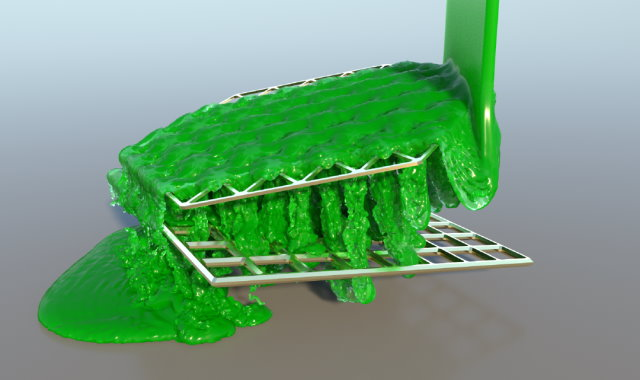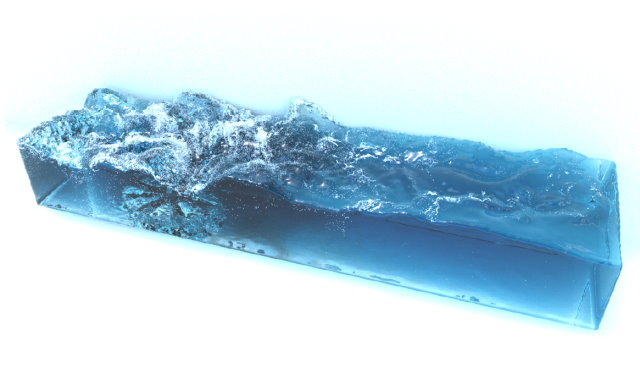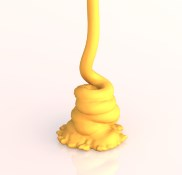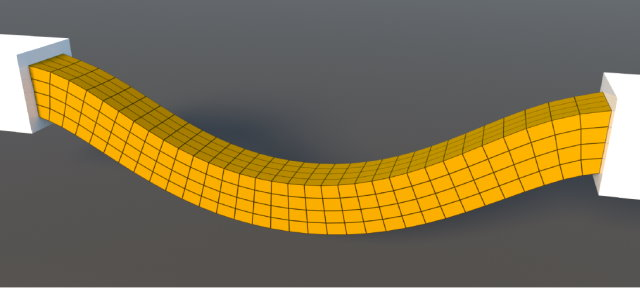# Physics Simulation in Visual Computing

SS 2023

Lecture

6 ECTS credits

### Contact:

bender@cs.rwth-aachen.de
Find a list of current courses on the Teaching page.
Course Dates:

### Room

Lecture Mon, 10:30 – 12:00, starting April 17 Room 5056 (2356|056)
Lecture/Exercise Thu, 10:30 - 12:00, starting April 06 AH VI (2356|051)

Physics simulation is an important research topic in visual computing. It has many applications ranging from such as virtual prototyping, training simulators, robotics, animation software for digital production including visual effects in film and animation movies, and computer games – just to mention a few.

This lecture gives an introduction to state-of-the-art simulation methods for deformable solids and fluids in the area of visual computing with a special focus on continuum mechanical approaches. Different popular discretization techniques will be discussed, namely the Finite Element Method (FEM) and the Smoothed Particle Hydrodynamics (SPH) formulation. All required mathematical foundations will be introduced in the lecture.In particular, we will consider the following topics:

• introduction in physics simulation
• mathematical foundations
• introduction of tensors
• numerical differentiation
• numerical integration
• simulation of deformable solids
• basics
• continuous model for deformable solids
• equation of motion
• finite element method (FEM)
• fluid simulation
• basics
• incompressible fluids
• Navier-Stokes equations
• Smoothed Particle Hydrodynamics (SPH)
• pressure solvers
• boundary handling
• viscosity
• surface tension
• vorticity